# pentropy

Spectral entropy of signal

## Syntax

``se = pentropy(xt)``
``se = pentropy(x,sampx)``
``se = pentropy(p,fp,tp)``
``se = pentropy(___,Name=Value)``
``[se,t] = pentropy(___)``
``pentropy(___)``

## Description

example

````se = pentropy(xt)` returns the Spectral Entropy of single-variable, single-column `timetable` `xt` as the `timetable` `se`. `pentropy` computes the spectrogram of `xt` using the default options of `pspectrum`.```

example

````se = pentropy(x,sampx)` returns the spectral entropy of vector `x`, sampled at rate or time interval `sampx`, as a vector. ```

example

````se = pentropy(p,fp,tp)` returns the spectral entropy using the power spectrogram `p`, along with spectrogram frequency and time vectors `fp` and `tp`. Use this syntax when you want to customize the options for `pspectrum`, rather than accept the default `pspectrum` options that `pentropy` applies.```

example

````se = pentropy(___,Name=Value)` specifies additional properties using name-value arguments. Options include instantaneous or whole-signal entropy, scaling by white noise entropy, frequency limits, and time limits. You can use `Name=Value` with any of the input arguments in previous syntaxes.```

example

````[se,t] = pentropy(___)` returns the spectral entropy `se` along with the time vector or `timetable` `t`. If `se` is a `timetable`, then `t` is equal to the row times of `timetable` `se`. This syntax does not apply if `Instantaneous` is set to `false`.```
````pentropy(___)` with no output arguments plots the spectral entropy against time. If `Instantaneous` is set to `false`, the function outputs the scalar value of the spectral entropy.```

## Examples

collapse all

Plot the spectral entropy of a signal expressed as a timetable and as a time series.

Generate a random series with normal distribution (white noise).

`xn = randn(1000,1);`

Create time vector `t` and convert to `duration` vector `tdur`. Combine `tdur` and `xn` in a timetable.

```fs = 10; ts = 1/fs; t = 0.1:ts:100; tdur = seconds(t); xt = timetable(tdur',xn);```

Plot the spectral entropy of the timetable `xt`.

```pentropy(xt) title('Spectral Entropy of White Noise Signal Timetable')```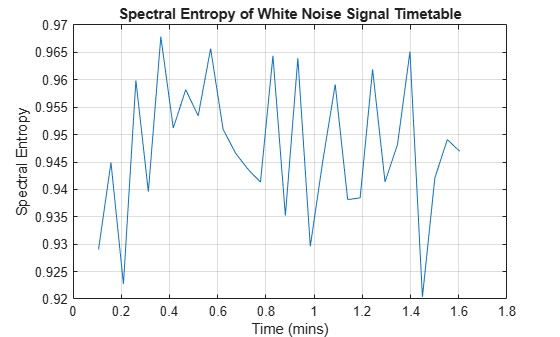Plot the spectral entropy of the signal, using time-point vector `t` and the form which returns `se` and associated time `te`. Match the x-axis units and grid to the `pentropy`-generated plots for comparison.

```[se,te] = pentropy(xn,t'); te_min = te/60; plot(te_min,se) title('Spectral Entropy of White Noise Signal Vector') xlabel('Time (mins)') ylabel('Spectral Entropy') grid on```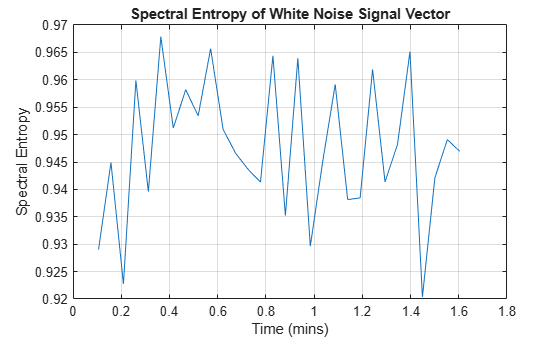Both yield the same result.

The second input argument for `pentropy` can represent either frequency or time. The software interprets according to the data type of the argument. Plot the spectral entropy of the signal, using sample rate scalar `fs` instead of time vector `t`.

```pentropy(xn,fs) title('Spectral Entropy of White Noise Signal Vector using Sample Rate')```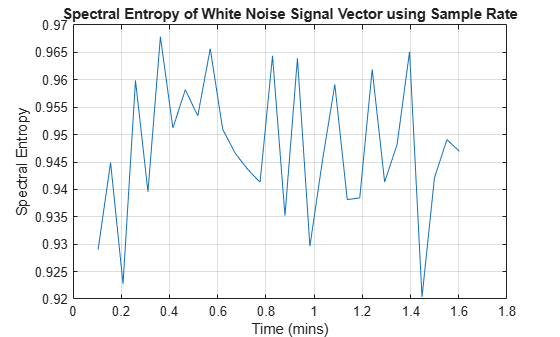This plot matches the previous plots.

Plot the spectral entropy of a speech signal and compare it to the original signal. Visualize the spectral entropy on a color map by first creating a power spectrogram, and then taking the spectral entropy of frequency bins within the bandwidth of speech.

Load the data `x`, which contains a two-channel recording of the word "Hello" embedded by low-level white noise. `x` consists of two columns representing the two channels. Use only the first channel.

Define the sample rate and the time vector. Augment the first channel of `x` with white noise to achieve a signal-to-noise ratio of about 5 to 1.

```load Hello x fs = 44100; t = 1/fs*(0:length(x)-1); x1 = x(:,1) + 0.01*randn(length(x),1);```

Find the spectral entropy. Visualize the data for the original signal and for the spectral entropy.

```[se,te] = pentropy(x1,fs); subplot(2,1,1) plot(t,x1) ylabel("Speech Signal") xlabel("Time") subplot(2,1,2) plot(te,se) ylabel("Spectral Entropy") xlabel("Time")```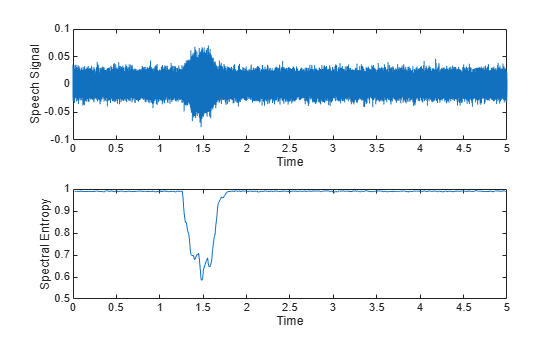The spectral entropy drops when "Hello" is spoken. This is because the signal spectrum has changed from almost a constant (white noise) to the distribution of a human voice. The human-voice distribution contains more information and has lower spectral entropy.

Compute the power spectrogram `p` of the original signal, returning frequency vector `fp` and time vector `tp` as well. For this case, specifying a frequency resolution of 20 Hz provides acceptable clarity in the result.

`[p,fp,tp] = pspectrum(x1,fs,"spectrogram",FrequencyResolution=20);`

The frequency vector of the power spectrogram goes to 22,050 Hz, but the range of interest with respect to speech is limited to the telephony bandwidth of 300–3400 Hz. Divide the data into five frequency bins by defining start and end points, and compute the spectral entropy for each bin.

```flow = [300 628 1064 1634 2394]; fup = [627 1060 1633 2393 3400]; se2 = zeros(length(flow),size(p,2)); for i = 1:length(flow) se2(i,:) = pentropy(p,fp,tp,FrequencyLimits=[flow(i) fup(i)]); end```

Visualize the data in a color map that shows ascending frequency bins, and compare with the original signal.

```figure subplot(2,1,1) plot(t,x1) xlabel("Time (seconds)") ylabel("Speech Signal") subplot(2,1,2) imagesc(tp,[],flip(se2)) % Flip se2 so its plot corresponds to the % ascending frequency bins. h = colorbar(gca,"NorthOutside"); ylabel(h,"Spectral Entropy") yticks(1:5) set(gca,YTickLabel=num2str((5:-1:1).')) % Label the ticks for the ascending bins. xlabel("Time (seconds)") ylabel("Frequency Bin")```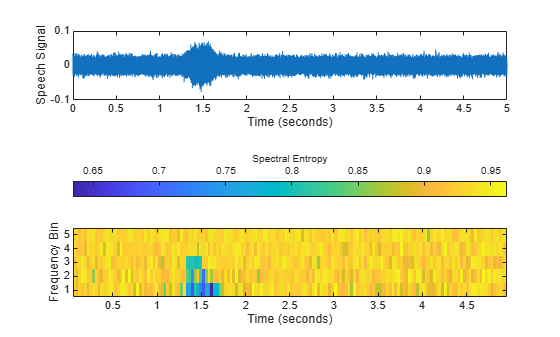Create a signal that combines white noise with a segment that consists of a sine wave. Use spectral entropy to detect the existence and position of the sine wave.

Generate and plot the signal, which contains three segments. The middle segment contains the sine wave along with white noise. The other two segments are pure white noise.

```fs = 100; t = 0:1/fs:10; sin_wave = 2*sin(2*pi*20*t')+randn(length(t),1); x = [randn(1000,1);sin_wave;randn(1000,1)]; t3 = 0:1/fs:30; plot(t3,x) title("Sine Wave in White Noise")```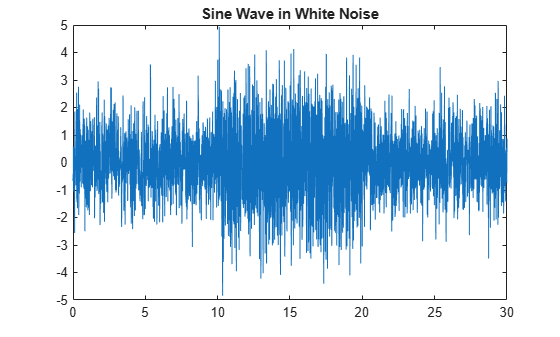Plot the spectral entropy.

```pentropy(x,fs) title("Spectral Entropy of Sine Wave in White Noise")```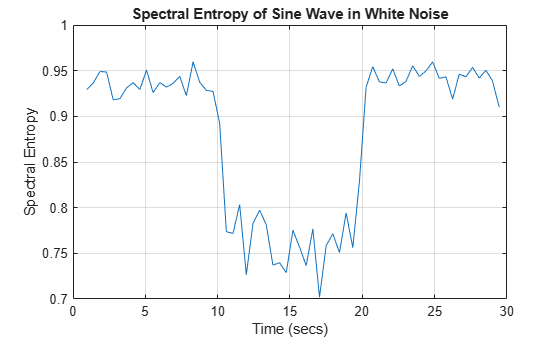The plot clearly differentiates the segment with the sine wave from the white-noise segments. This is because the sine wave contains information. Pure white noise has the highest spectral entropy.

The default for `pentropy` is to return or plot the instantaneous spectral entropy for each time point, as the previous plot displays. You can also distill the spectral entropy information into a single number that represents the entire signal by setting `Instantaneous` to `false`. Use the form that returns the spectral entropy value if you want to directly use the result in other calculations. Otherwise, `pentropy` returns the spectral entropy in `ans`.

`se = pentropy(x,fs,Instantaneous=false)`
```se = 0.9033 ```

A single number characterizes the spectral entropy, and therefore the information content, of the signal. You can use this number to efficiently compare this signal with other signals.

## Input Arguments

collapse all

Signal timetable from which `pentropy` returns the spectral entropy `se`, specified as a `timetable` that contains a single variable with a single column. `xt` must contain increasing, finite row times. If the `xt` `timetable` has missing or duplicate time points, you can fix it using the tips in Clean Timetable with Missing, Duplicate, or Nonuniform Times. `xt` can be nonuniformly sampled, with the `pspectrum` constraint that the median time interval and the mean time interval must obey:

For an example, see Plot Spectral Entropy of Signal.

Time-series signal from which `pentropy` returns the spectral entropy `se`, specified as a vector.

Sample rate or sample time, specified as one of the following:

When `sampx` represents a time vector, time samples can be nonuniform, with the `pspectrum` constraint that the median time interval and the mean time interval must obey:

For an example, see Plot Spectral Entropy of Signal.

Power spectrogram or spectrum of a signal, specified as a matrix (spectrogram) or a column vector (spectrum). If you specify `p`, then `pentropy` uses `p` rather than generate its own spectrogram or power spectrogram. `fp` and `tp`, which provide the frequency and time information, must accompany `p`. Each element of `p` at the i'th row and the j'th column represents the signal power at the frequency bin centered at `fp`(i) and the time instance `tp`(j).

For an example, see Plot Spectral Entropy of Speech Signal.

Frequencies for spectrogram or power spectrogram `p` when `p` is supplied explicitly to `pentropy`, specified as a vector in hertz. The length of `fp` must be equal to the number of rows in `s`.

Time information for power spectrogram or spectrum `p` when `p` is supplied explicitly to `pentropy`, specified as one of the following:

• Vector of time points, whose data type can be numeric, `duration`, or `datetime`. The length of vector `tp` must be equal to the number of columns in `p`.

• `duration` scalar that represents the time interval in `p`. The scalar form of `tp` can be used only when `p` is a power spectrogram matrix.

• For the special case where `p` is a column vector (power spectrum), `tp` can be a numeric, `duration`, or `datetime` scalar representing the time point of the spectrum.

For the special case where `p` is a column vector (power spectrum), `tp` can be a single/double/`duration`/`datetime` scalar representing the time point of the spectrum.

### Name-Value Arguments

Specify optional pairs of arguments as `Name1=Value1,...,NameN=ValueN`, where `Name` is the argument name and `Value` is the corresponding value. Name-value arguments must appear after other arguments, but the order of the pairs does not matter.

Before R2021a, use commas to separate each name and value, and enclose `Name` in quotes.

Example: `"Instantaneous",false,"FrequencyLimits",[25 50]` computes the scalar spectral entropy representing the portion of the signal ranging from 25 Hz to 50 Hz.

Instantaneous time series option, specified as a logical.

• If `Instantaneous` is `true`, then `pentropy` returns the instantaneous spectral entropy as a time-series vector.

• If `Instantaneous` is `false`, then `pentropy` returns the spectral entropy value of the whole signal or spectrum as a scalar.

For an example, see Use Spectral Entropy to Detect Sine Wave in White Noise.

Scale by white noise option, specified as a logical. Scaling by white noise — or log2n, where n is the number of frequency points — is equivalent to normalizing in Spectral Entropy. It allows you to perform a direct comparison on signals of different length.

• If `Scaled` is `true`, then `pentropy` returns the spectral entropy scaled by the spectral entropy of the corresponding white noise.

• If `Scaled` is `false`, then `pentropy` does not scale the spectral entropy.

Frequency limits to use, specified as a two-element vector containing lower and upper bounds f1 and f2 in hertz. The default is [0 `sampfreq`/2], where `sampfreq` is the sample rate in hertz that `pentropy` derives from `sampx`.

This specification allows you to exclude a band of data at either end of the spectral range.

For an example, see Plot Spectral Entropy of Speech Signal.

Time limits, specified as a two-element vector containing lower and upper bounds t1 and t2 in the same units as the sample time provided in `sampx`, and of the data types:

• Numeric or `duration` when `sampx` is numeric or duration

• Numeric, `duration`, or `datetime` when `sampx` is `datetime`

This specification allows you to extract a time segment of data from the full timespan.

## Output Arguments

collapse all

Spectral Entropy, returned as a `timetable` if the input signal is `timetable` `xt`, and as a double vector if the input signal is time series `x`.

Time values associated with `se`, returned in the same form as the time in `se`. This argument does not apply if `Instantaneous` is set to `false`.

For an example, see Plot Spectral Entropy of Signal.

collapse all

### Spectral Entropy

The spectral entropy (SE) of a signal is a measure of its spectral power distribution. The concept is based on the Shannon entropy, or information entropy, in information theory. The SE treats the signal's normalized power distribution in the frequency domain as a probability distribution, and calculates the Shannon entropy of it. The Shannon entropy in this context is the spectral entropy of the signal. This property can be useful for feature extraction in fault detection and diagnosis , . SE is also widely used as a feature in speech recognition  and biomedical signal processing .

The equations for spectral entropy arise from the equations for the power spectrum and probability distribution for a signal. For a signal x(n), the power spectrum is S(m) = |X(m)|2, where X(m) is the discrete Fourier transform of x(n). The probability distribution P(m) is then:

`$P\left(m\right)=\frac{S\left(m\right)}{{\sum }_{i}S\left(i\right)}\text{.}$`

The spectral entropy H follows as:

`$H=-\sum _{m=1}^{N}P\left(m\right){\mathrm{log}}_{2}P\left(m\right)\text{.}$`

Normalizing:

`${H}_{n}=-\frac{\sum _{m=1}^{N}P\left(m\right){\mathrm{log}}_{2}P\left(m\right)}{{\mathrm{log}}_{2}N}\text{,}$`

where N is the total frequency points. The denominator, log2N represents the maximal spectral entropy of white noise, uniformly distributed in the frequency domain.

If a time-frequency power spectrogram S(t,f) is known, then the probability distribution becomes:

`$P\left(m\right)=\frac{{\sum }_{t}S\left(t,m\right)}{{\sum }_{f}{\sum }_{t}S\left(t,f\right)}.$`

Spectral entropy is still:

`$H=-\sum _{m=1}^{N}P\left(m\right){\mathrm{log}}_{2}P\left(m\right)\text{.}$`

To compute the instantaneous spectral entropy given a time-frequency power spectrogram S(t,f), the probability distribution at time t is:

`$P\left(t,m\right)=\frac{S\left(t,m\right)}{{\sum }_{f}S\left(t,f\right)}.$`

Then the spectral entropy at time t is:

`$H\left(t\right)=-\sum _{m=1}^{N}P\left(t,m\right){\mathrm{log}}_{2}P\left(t,m\right).$`

 Pan, Y. N., J. Chen, and X. L. Li. "Spectral Entropy: A Complementary Index for Rolling Element Bearing Performance Degradation Assessment." Proceedings of the Institution of Mechanical Engineers, Part C: Journal of Mechanical Engineering Science. Vol. 223, Issue 5, 2009, pp. 1223–1231.

 Sharma, V., and A. Parey. "A Review of Gear Fault Diagnosis Using Various Condition Indicators." Procedia Engineering. Vol. 144, 2016, pp. 253–263.

 Shen, J., J. Hung, and L. Lee. "Robust Entropy-Based Endpoint Detection for Speech Recognition in Noisy Environments." ICSLP. Vol. 98, November 1998.

 Vakkuri, A., A. Yli‐Hankala, P. Talja, S. Mustola, H. Tolvanen‐Laakso, T. Sampson, and H. Viertiö‐Oja. "Time‐Frequency Balanced Spectral Entropy as a Measure of Anesthetic Drug Effect in Central Nervous System during Sevoflurane, Propofol, and Thiopental Anesthesia." Acta Anaesthesiologica Scandinavica. Vol. 48, Number 2, 2004, pp. 145–153.